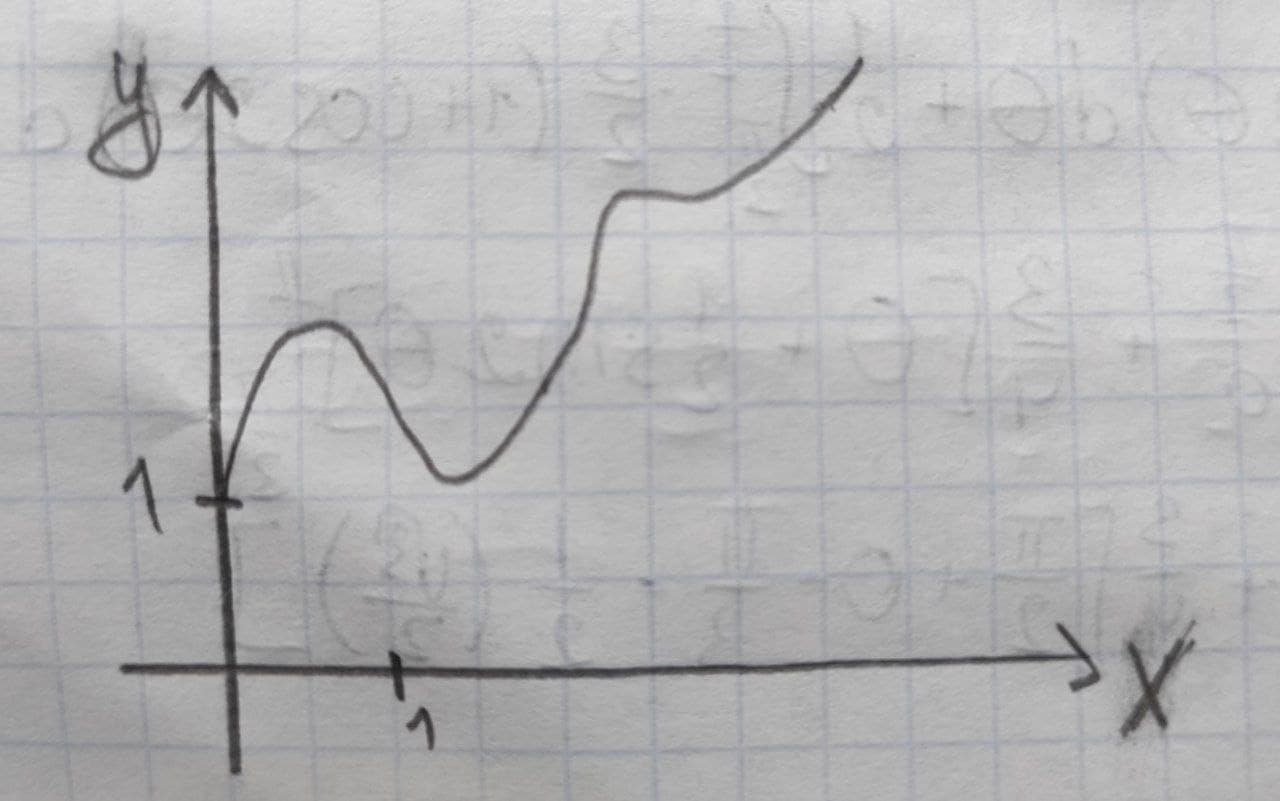# Get help with parametric equations

Recent questions in Parametric equationsFalak Kinney 2021-09-15 Answered

### Find sets of parametric equations and symmetric equations of the line that passes through the given point and is parallel to the given vector or line. (For each line, write the direction numbers as integers.) Point: (-3, 4, 5) Parallel to: $$\displaystyle\frac{{{x}-{1}}}{{2}}=\frac{{{y}+{1}}}{ -{{3}}}={z}-{5}$$ (a) Parametric equations (b) Symmetric equationsTahmid Knox 2021-09-15 Answered

### Given the points (2,6) and (6,10), find at least three equations from exponential function that pass through these pointJoni Kenny 2021-09-15 Answered

### For the given polar equation, write an equivalent rectangular equation $$\displaystyle{r}{\cos{\theta}}={13}$$UkusakazaL 2021-09-14 Answered

### Replace the polar equations with equivalent Cartesian equations. Then describe or identify the graph. $$\displaystyle{r}^{{{2}}}=-{4}{r}{\cos{\theta}}$$abondantQ 2021-09-14 Answered

### Convert the point from rectangular coordinates to spherical coordinates. $$\displaystyle{\left(-{3},-{3},\sqrt{{{2}}}\right)}$$coexpennan 2021-09-14 Answered

### Find parametric equations for x, y, and z in terms of the polar coordinates r and $$\displaystyle\theta$$ to determine the points on the portion of the paraboloid $$x + y + z = 5$$ that is on or above the plane $$z=4$$Tyra 2021-09-14 Answered

### Find the length of the parametric curve defined over the given interval $$\displaystyle{x}={3}{t}-{6},{y}={6}{t}+{1},{0}\le{t}\le{1}$$Zoe Oneal 2021-09-13 Answered

### A vector-valued function $$r(t)$$ with its defining parametric equations is given by the following. $$x = f(t)$$ $$y = g(t)$$ $$r(t) = ft(i) + g(t)j$$ Now find the vector-valued function $$r(t)$$ with the given parametric equations.Falak Kinney 2021-09-12 Answered

### Polar coordinates of point P are given. Find all of its polar coordinates. $$\displaystyle{P}={\left({1},-\frac{\pi}{{4}}\right)}$$Wribreeminsl 2021-09-12 Answered

### Find the area of the largest rectangle that can be inscribed in the ellipse $$\displaystyle{\frac{{{x}^{{{2}}}}}{{{a}^{{{2}}}}}}+{\frac{{{y}^{{{2}}}}}{{{b}^{{{2}}}}}}={1}$$OlmekinjP 2021-09-12 Answered

### Surface s is a part of the paraboloid $$\displaystyle{z}={4}-{x}^{{2}}-{y}^{{2}}$$ that lies above the plane $$z=0$$.$$(6+7+7=20pt)$$ a) Find the parametric equation $$\displaystyle\vec{{r}}{\left({u},{v}\right)}$$ of the surface with polar coordinates $$\displaystyle{x}={u}{\cos{{\left({v}\right)}}},{y}={u}{\sin{{\left({v}\right)}}}$$ and find the domain D for u and v. b) Find $$\displaystyle\vec{{r}}_{{u}},\vec{{r}}_{{v}},$$ and $$\displaystyle\vec{{r}}_{{u}}\cdot\vec{{r}}_{{v}}$$. c) Find the area of the surfaceNann 2021-09-12 Answered

### Determine the x-y coordinates of the points where the following parametric equations will have horizontal or vertical tangents $$\displaystyle{x}={t}^{{3}}-{3}{t}$$ $$\displaystyle{y}={3}{t}^{{2}}-{9}$$UkusakazaL 2021-09-11 Answered

### Express the point with cartesian coordinates Q(3,-1) in polar formWierzycaz 2021-09-11 Answered

### $$x = t− \sin t$$ $$y = 1 − \cos t −$$ curve given by the parametric equation $$\displaystyle=\frac{\pi}{{3}}$$ in point Find the equation of the tangent lineopatovaL 2021-09-10 Answered

### Write the equation of the line tangent to $$\displaystyle{f{{\left({x}\right)}}}={\tan{{x}}}+{3}$$ at $$\displaystyle{\left(\frac{\pi}{{4}}.{4}\right)}$$Chaya Galloway 2021-09-10 Answered

### Use the given graph of f over the interval (0,7) to find the following.(a) The open intervals on which f is increasing. (Enter your answer using interval notation.) (b) The open intervals on which f is decreasing. (Enter your answer using interval notation.) (c) The open intervals on which f is concave upward. (Enter your answer using interval notation.) (d) The open intervals on which f is concave downward. (Enter your answer using interval notation.) (e) The coordinates of the points of inflection. (x,y)=( ) (smallest x-value) (x,y)=( ) (x,y)=( ) (largest x-value)Anish Buchanan 2021-09-10 Answered

### Determine the line integral along the curve C from A to B. Find the parametric form of the curve C Use the vector field: $$\overrightarrow{F}=[-bx\ cy]$$ Use the following values: $$a=5,\ b=2,\ c=3$$Tolnaio 2021-09-10 Answered

### Write an equations in indicated coordinate system. Also write a parametric equation and a vector equation for each of the following equations. 1) $$\displaystyle{\frac{{{x}-{1}}}{{{2}}}}={\frac{{{y}-{5}}}{{{3}}}}={\frac{{{z}-{2}}}{{{4}}}}$$ cylindrical coordinates, sherical coordinates 2) $$\displaystyle{3}{x}+{4}{y}+{5}{z}={6}$$ cylindrical coordinates, sherical coordinates 3) $$\displaystyle{x}^{{2}}+{y}^{{2}}+{z}^{{2}}={100}$$ cylindrical coordinates, sherical coordinates 4) $$\displaystyle{x}^{{2}}+{y}^{{2}}={100}$$ cylindrical coordinates, sherical coordinatesMaiclubk 2021-09-09 Answered

### Find the point(s) of intersection of the following two parametric curves, by first eliminating the parameter, then solving the system of equations. $$\displaystyle{x}={t}+{5},{y}={t}^{{{2}}}{\quad\text{and}\quad}{x}={\frac{{{1}}}{{{16}}}}{t},{y}={t}$$ a. (25, 400) b. (1, 16) c. (400, 16) d. A and B e. A and CNann 2021-09-09 Answered

...